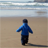Announcements
[WEBINAR] Accenture & Qlik: Accelerating BI Migration to SaaS with Qlik on Dec 13th: REGISTER
cancel
Showing results for
Did you mean:Creator III

## If condition with multiple criteria using AND, also value if TRUE is aggregation

I'm trying to write a condition on some of my bar charts that would be equivalent to SUMIFS or COUNTIFS variations in Excel. If my IF condition meets two criteria (AND) then I want two values aggregated (summed)

here's what I have written:

=if(FCRA_dummy=1 AND [Not_e-Oscar_Dum]=1, count(Pkey)) + count([ACDV Control Number])

IF both of my dummies are "1", I just want a count of a distinct column in my data, then sum that with another count of a distinct column in my data. I am not getting an error with this expression, but it isn't showing any data, and I know that's not true. I'm sure there is a simple solution to this, or maybe even a function built in to QV.. Any ideas on why this isn't working?

1 Solution

Accepted SolutionsMVP

=If(FCRA_dummy = 1 and [Not_e-Oscar_Dum] = 1, Count(Pkey) + Count([ACDV Control Number]))

or

=Count({<FCRA_dummy = {1}, [Not_e-Oscar_Dum] = {1}>} Pkey) + Count({<FCRA_dummy = {1}, [Not_e-Oscar_Dum] = {1}>}[ACDV Control Number])

2 RepliesMVP

=If(FCRA_dummy = 1 and [Not_e-Oscar_Dum] = 1, Count(Pkey) + Count([ACDV Control Number]))

or

=Count({<FCRA_dummy = {1}, [Not_e-Oscar_Dum] = {1}>} Pkey) + Count({<FCRA_dummy = {1}, [Not_e-Oscar_Dum] = {1}>}[ACDV Control Number])Creator III
Author

I did indeed use Set Analysis to solve this issue. ThanksCommunity Browser